Properties
Translations
Rotations
Reflections
Random Trivia
100

Define congruence.

The same shape, same size?

100

What is another word for translating?

Sliding or shifting

100

What word describes rotating?

Turning

100

What is another word for reflection?

Flipping, mirroring

100

What temperature does water freeze at?

0 degrees Celsius, 32 degrees Farenheit

200

These are the types of rigid transformations.

Reflections, translations, and reflections

200

Adding to the x-coordinate moves a figure in this direction on a graph.

Right

200

Making both coordinates negative rotates the figure how many degrees?

180o

200

True or false, mirror images are ALWAYS congruent?

True

200

A triangle with 3 unequal sides is called what?

A scalene triangle

300

The picture below shows this type of transformation.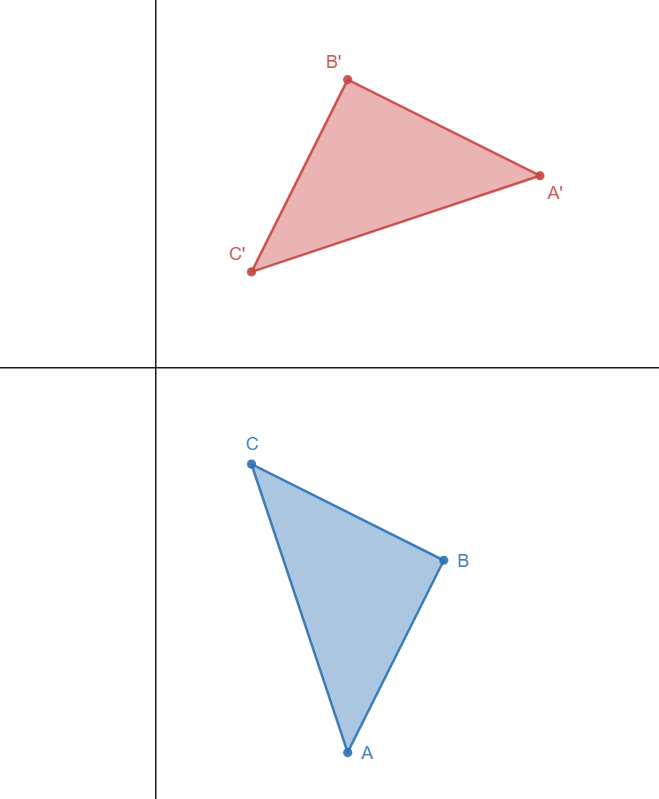Rotational symmetry

300

What are the coordinates of the image when the pre-image (1, 6) is translated to the left 3 units and down 1 unit?

(-2, 5)

300

The green segment below has been rotated 90o in what direction?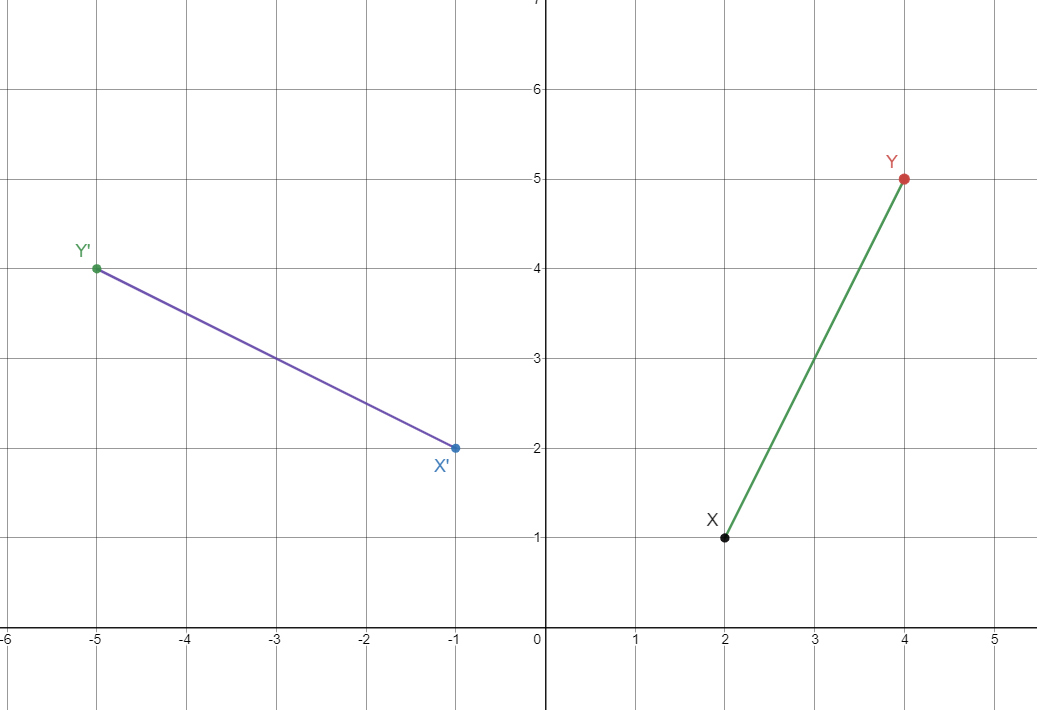Counterclockwise

300

Which coordinate changes when a figure is reflected over the x-axis?

The y-coordinate

300

Taste, smell, touch, hearing, & sight

400

This notation is how we differentiate the preimage from the image

Prime notation, ex. A -> A'

400

What are the coordinates of the image when the coordinates of the pre image (-1,-5) is translated 4 units to the left and 6 units up?

(-5,1)

400

Name the coordinates for M' after the figure below is rotated 180o clockwise.(-6, -6)

400

The green figure has been reflected over which line?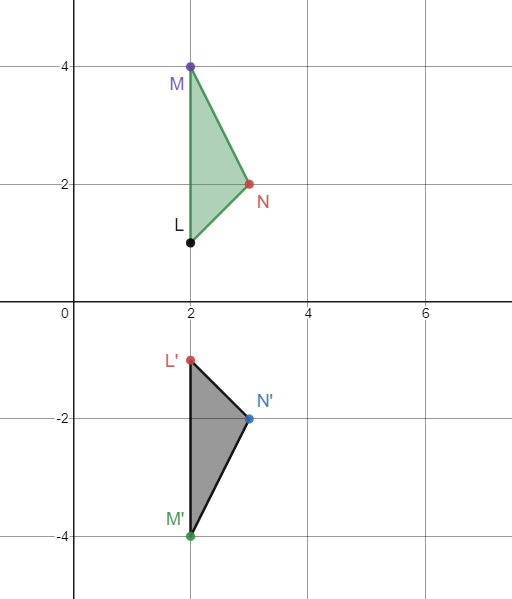The x-axis

400

How many bones are in the human body?

206

500

Which colored figure is the rotated image in the following reflection: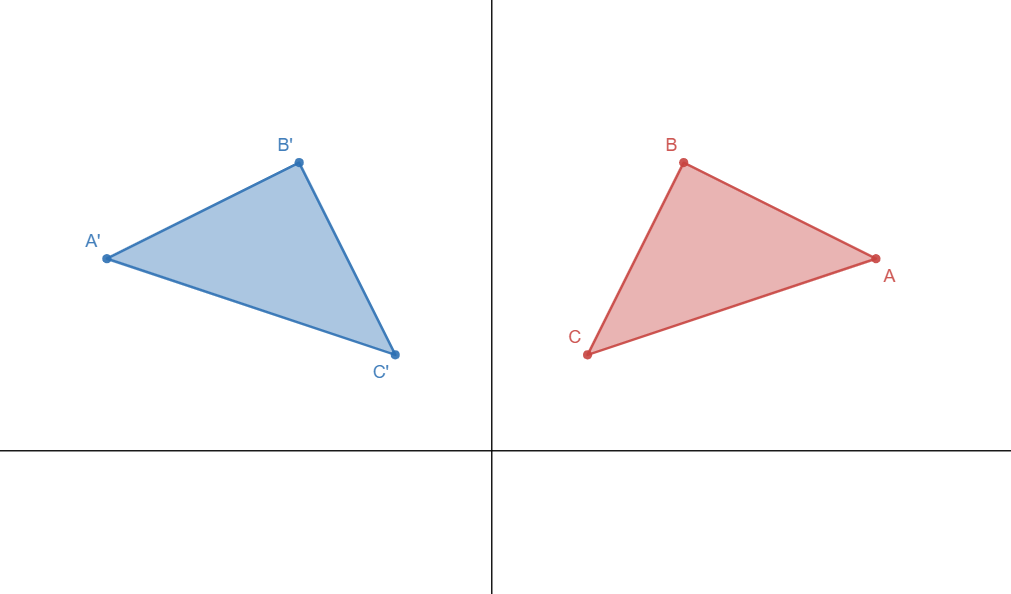The blue figure

500

The green shape is translated in which directions and how many units?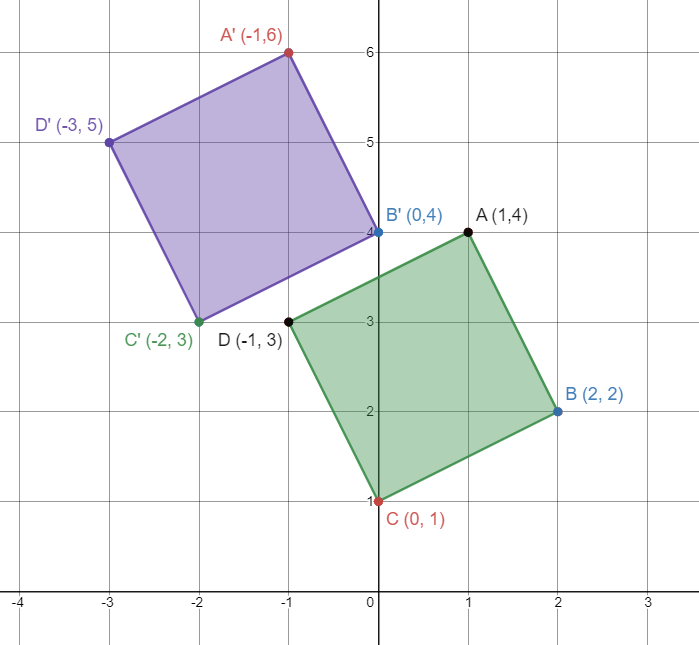Left two units, up two units

500

What clockwise rotation is the same as rotating 90o counterclockwise.

270o clockwise

500

Name the coordinates of A' after the figure below has been reflected over the line x=4.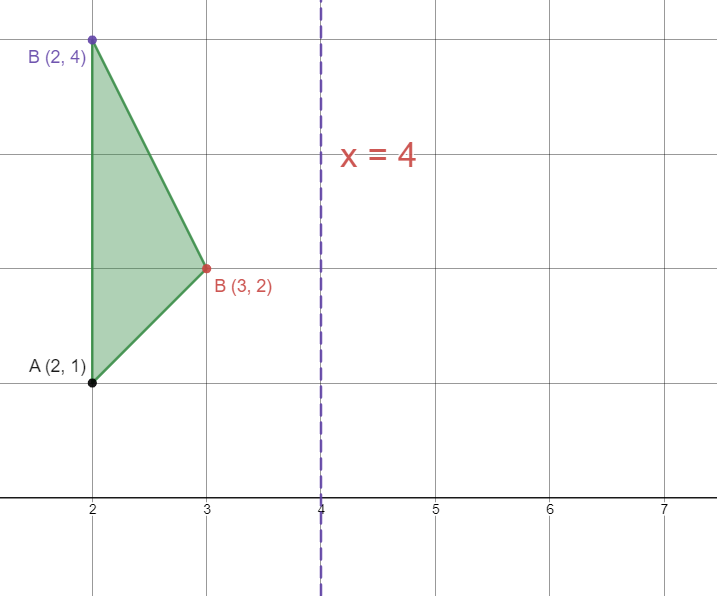(6, 1)

500

Who lives in a trashcan on Sesame Street?

Oscar the Grouch

Click to zoom